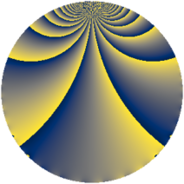# Properties

 Label 2760.2.fLevel $2760$ Weight $2$ Character orbit 2760.f Rep. character $\chi_{2760}(829,\cdot)$ Character field $\Q$ Dimension $264$ Sturm bound $1152$

# Related objects

## Defining parameters

 Level: $$N$$ $$=$$ $$2760 = 2^{3} \cdot 3 \cdot 5 \cdot 23$$ Weight: $$k$$ $$=$$ $$2$$ Character orbit: $$[\chi]$$ $$=$$ 2760.f (of order $$2$$ and degree $$1$$) Character conductor: $$\operatorname{cond}(\chi)$$ $$=$$ $$40$$ Character field: $$\Q$$ Sturm bound: $$1152$$

## Dimensions

The following table gives the dimensions of various subspaces of $$M_{2}(2760, [\chi])$$.

Total New Old
Modular forms 584 264 320
Cusp forms 568 264 304
Eisenstein series 16 0 16

## Trace form

 $$264q + 264q^{9} + O(q^{10})$$ $$264q + 264q^{9} + 20q^{10} + 40q^{14} - 12q^{20} - 8q^{25} + 16q^{26} - 8q^{30} + 48q^{31} - 16q^{34} + 32q^{39} - 20q^{40} + 16q^{41} - 40q^{44} - 264q^{49} - 16q^{50} + 32q^{55} + 16q^{56} + 24q^{60} + 48q^{64} + 48q^{65} - 40q^{66} - 32q^{70} - 64q^{71} - 88q^{74} + 48q^{76} + 28q^{80} + 264q^{81} - 24q^{84} - 16q^{86} + 80q^{89} + 20q^{90} - 64q^{94} - 48q^{95} + O(q^{100})$$

## Decomposition of $$S_{2}^{\mathrm{new}}(2760, [\chi])$$ into newform subspaces

The newforms in this space have not yet been added to the LMFDB.

## Decomposition of $$S_{2}^{\mathrm{old}}(2760, [\chi])$$ into lower level spaces

$$S_{2}^{\mathrm{old}}(2760, [\chi]) \cong$$ $$S_{2}^{\mathrm{new}}(40, [\chi])$$$$^{\oplus 4}$$$$\oplus$$$$S_{2}^{\mathrm{new}}(120, [\chi])$$$$^{\oplus 2}$$$$\oplus$$$$S_{2}^{\mathrm{new}}(920, [\chi])$$$$^{\oplus 2}$$